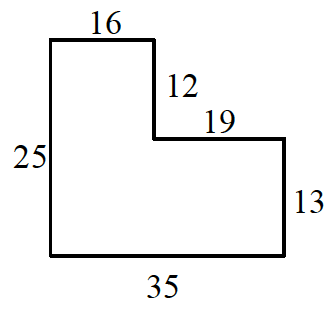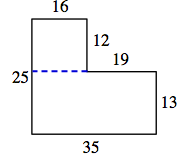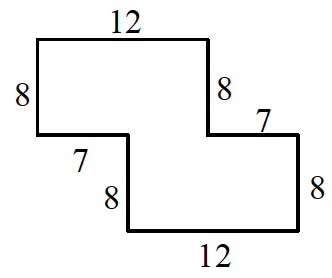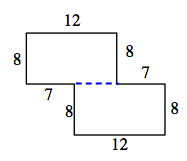### Home > CCAA > Chapter 2 Unit 4 > Lesson CC3: 2.1.1 > Problem2-8

2-8.

Copy the following figures onto your paper. Then find the area and perimeter of each shape. Assume that all corners are right angles. Show all work.

1.To find the area, separate the shape into two boxes, find the area of the two sections, and then add them together for the total area.

$16\times12=192$
$13 \times 35 = 455$
area $=192+455$

area $=647$ units2

To find the perimeter (the distance around a shape) add up all of the sides of the shape.

perimeter $=16+12+19+13+35+25$

perimeter $=120$ units

1.To find the area and perimeter of the shape in part (b), follow the same strategy outlined in part (a).